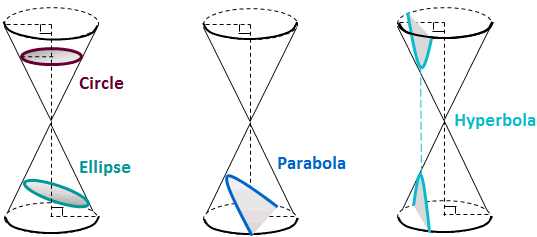# Science Bytes: Newton’s “Power Curves”

Every student who grinds through calculus will, at some point, come face to face with the logarithm function (the “log function” for short), an object of enormous scientific importance. Yet, the log function is so ubiquitous and so easy to compute that we hardly notice its underlying beauty. Which reminds me of the old joke about the fish who ask his friend, “Aren’t you grateful for water?” to which the other fish says, “What water?”

To appreciate the beauty of the log function, we go back to 17th century. It is the winter of 1664-65 and we are in Cambridge University, where a young Issac Newton, then only twenty-two, was thinking deeply about curves and calculus.

Then, an epiphany occurred. Newton discovered that any curve (more precisely, the polynomial equation that describes that curve) can be expressed as a series of power functions. The simplest power function – one with a single unknown variable (call it x) – is an equation that adds up infinitely many quantities involving x. Through a process of inspired guesswork and intuition, Newton discovered that the area of a circular segment could be expressed by the following power series:$\displaystyle{A(x) =x- \frac{1}{6} x^3-\frac{1}{40} x^5-\frac{1}{112} x^7-\frac{5}{1152} x^9-... }$

As for how those peculiar terms came from, well’s that was Newton’s secret sauce (look up any good calculus textbook for the derivations if interested).

The ever-ambitious Newton went on to explore other curves such as the hyperbola, and it is this curve that led him to the log function (Newton called it the hyperbolic logarithm).The hyperbola belongs to the same class as the circle, ellipse and parabola. All are examples of “conic sections”.

The equation of a hyperbola is$\displaystyle{\frac{1}{1+x} }$. See footnote 1 for details. Newton showed that this equation can be expressed by the following power series:$\displaystyle{\frac{1}{1+x} = 1-x+x^2-x^3+x^4-x^5+ ... }$

This rather neat series in turn led him to a power series for the area of a segment under the hyperbola from 0 to x (whatever x is), which is none other than natural logarithm function: ln(x):$\displaystyle{ \ln(x) = x-\frac{1}{2}x^2 + \frac{1}{3}x^3 -\frac{1}{4}x^4 + \frac{1}{5}x^5 + ... }$

If you are interested in the proof (not too hard), see footnote 2. For now, let’s just stand back and take in the harmonious beauty of this expression. Newton’s power series expression is a tour de force, impressive both for its analytic beauty and practicality. The beauty is plain to see: every term after x is followed by a term whose exponent is the exactly the same as the denominator in the fraction. For example, ignoring the + and – signs, the second term is$\frac{1}{2}x^2$, the third is$\frac{1}{3}x^3$ and so on, continuing indefinitely to infinity. Add in the alternating signs, and you can imagine a “sea” of of rising and falling waves. As for practicality, the expression allows the calculation of ln(x) to be speeded up enormously. This excited Newton greatly. It ought to excite us too.

There’s more. Centuries ago, the Greek mathematician Archimedes had derived the power series for a segment (the quadrature) of a parabola:$\displaystyle {\frac{4}{3} = 1 + \frac{1}{4} + \frac{1}{16} + \frac{1}{64} + ... }$

Plug x = -1/4 into Newton’s series, and it becomes Archimedes’s. In that sense, Newton’s expression is more general, adding another feather in the cap to Newton’s genius .

Notes:

 The hyperbola belongs to the same class with the circle, ellipse, and parabola. They are known as “conic sections” because they can be generated by intersecting a cone and a plane, and all have second degree equations. The equation$y= \frac{1}{1+x}$ is a hyperbola even though it doesn’t look like one. You probably learned that a hyperbola has the standard form of:$\frac{(1+x)^2}{a^2} - \frac{y^2}{b^2} = 1$, which is a second degree equation in two variables). The reason that y=1/1+x doesn’t look like that is because it has been rotated 45 degrees from the standard position. But it is really the hyperbola:$\frac{(1+x)^2}{2} - \frac{y^2}{2} = 1$ and it is centered at the origin with foci on the x-axis. It is symmetric with respect to the x-axis so it “opens” to the left and right. If you take this hyperbola and rotate it 45 degrees counterclockwise, you can show that the equation becomes$(1+x)y = 1$, which can be written$y = \frac{1}{1+x}$. (The “cross term” indicates it’s been rotated).

 I said no derivations, but I could not resist for ln(x) which have a beauty of its own. So, for nerds, below are the basic steps Newton used to proof the power series representation of ln(x).

 The method of power series is unquestionably one of Newton’s crowning achievements. Newton saw calculus as the algebraic analogue of arithmetic with infinite decimals, and he wrote his results in (1671; Treatise on the Method of Series and Fluxions). Being reclusive and painfully sensitive to criticism, he wanted to keep his secret fountain to himself, so this work didn’t see the light of day during his lifetime. It was published only in 1736, nine years after his death.